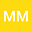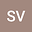Finite time collapse in chemotaxis systems with logistic-type superlinear source
••• Monica Marras,
• Stella Vernier-Piro
Monica Marras
University of Cagliari
Author ProfileStella Vernier-Piro
Universita di Cagliari
Author Profile## Abstract

We consider the following quasilinear Keller-Segel system \begin{equation*} \left\{ \begin{array}{l} \begin{aligned} u_t = \Delta u - \nabla (u \nabla v) + g(u), \quad &(x,t)\in \Omega \times [0,T_{max}) ,\\[6pt] 0= \Delta v - v + u, \qquad \quad &(x,t)\in \Omega \times [0,T_{max}), \\[6pt] \end{aligned} \end{array} \right. \end{equation*} on a ball $\Omega \equiv B_R(0)\subset\mathbb{R}^n$, $n\geq 3$, $R>0$, under homogeneous Neumann boundary conditions and non negative initial data. The source term $g(u)$ is superlinear and of logistic type i.e. $g(u)=\lambda u - \mu u^k,\ k>1, \ \mu >0$, $\lambda \in \mathbb{R}$ and $T_{max}$ is the blow-up time.\\ The solution $(u,v)$ may or may not blow up in finite time. Under suitable conditions on data, we prove that the function $u$, which blows up in $L^{\infty} (\Omega)$-norm \cite{W}, blows up also in $L^p(\Omega)$-norm for some $p>1$. Moreover a lower bound of the lifespan (or blow-up time when it is finite) $T_{max}$ is derived. \\ In addition, if $\Omega \subset \mathbb{R}^3$ a lower bound of $T_{max}$ is explicitly computable.

#### Peer review status:ACCEPTED

24 Feb 2020Submitted to Mathematical Methods in the Applied Sciences
28 Feb 2020Submission Checks Completed
28 Feb 2020Assigned to Editor
04 Mar 2020Reviewer(s) Assigned
16 Jun 2020Review(s) Completed, Editorial Evaluation Pending
16 Jun 2020Editorial Decision: Revise Minor